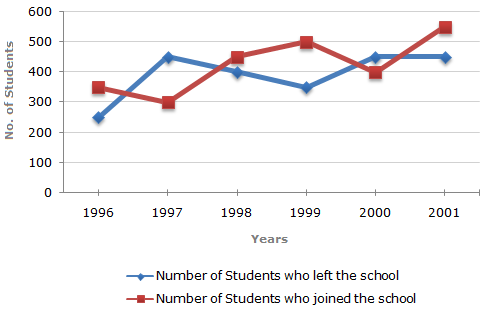### Line Chart - 6

Study the following line graph which gives the number of students who joined and left the school in the beginning of year for six years, from 1996 to 2001.

Initial Strength of school in 1995 = 3000.1.  The ratio of the least number of students who joined the school to the maximum number of students who left the school in any of the years during the given period is?

 A. 7 : 9 B. 4 : 5 C. 3 : 4 D. 9 : 11 E. 2 : 3
2.  The number of students studying in the school during 1999 was?

 A. 2950 B. 3000 C. 3100 D. 3150 E. 3200
3.  The strength of school increased/decreased from 1997 to 1998 by approximately what percent?

 A. 1.2% B. 1.7% C. 2.1% D. 2.4% E. 2.6%
4.  The number of students studying in the school in 1998 was what percent of the number of students studying in the school in 2001?

 A. 92.13% B. 93.75% C. 96.88% D. 97.25% E. 99%
5.  For which year, the percentage rise/fall in the number of students who left the school compared to the previous year is maximum?

 A. 1997 B. 1998 C. 1999 D. 2000 E. 2001